# Math in Focus Grade 5 Chapter 15 Practice 3 Answer Key Nets and Surface Area

This handy Math in Focus Grade 5 Workbook Answer Key Chapter 15 Practice 3 Nets and Surface Area provides detailed solutions for the textbook questions.

## Math in Focus Grade 5 Chapter 15 Practice 3 Answer Key Nets and Surface Area

Find the surface area of each cube.

Example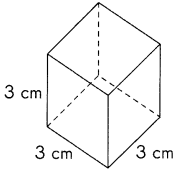3 × 3 = 9
6 × 9 = 54
Surface area of cube = 54 cm2

Question 1.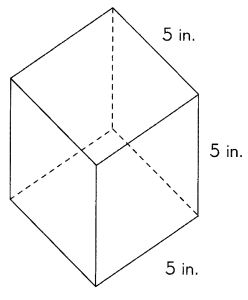S.A = 150 in.2,

Explanation:
As we know surface area (S.A) of cube is 6 X (side X side),
Given cube has 5 in side therefore S.A = 6 X (5 in X 5 in) =
6 X (25 in.2) = 150 in.2.

Question 2.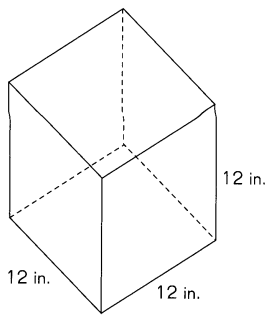S.A = 864 in.2,

Explanation:
As we know surface area (S.A) of cube is 6 X (side X side),
Given cube has 12 in side therefore S.A = 6 X (12 in X 12 in) =
6 X (144 in.2) = 864 in.2.

Question 3.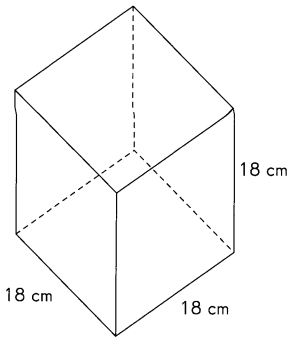S.A = 1924 cm2,

Explanation:
As we know surface area (S.A) of cube is 6 X (side X side),
Given cube has 18 in side therefore S.A = 6 X (18 cm X 18 cm) =
6 X (324 cm2) = 1924 cm2.

Find the surface area of each rectangular prism.

Example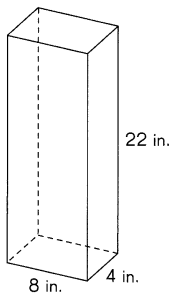2 × 8 × 4 = 64
2 × 22 × 4 = 176
2 × 22 × 8= 352
64 + 176 + 352 = 592
Surface area of rectangular prism = 592 in.2

Question 4.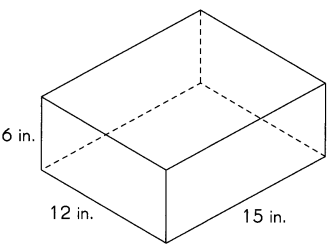The surface area of rectangular prism is 684 in.2,

Explanation:
As the surface area of rectangular prism is
2 X [(width X length) + (height X length) + (height X width)],
= 2 X [(12 in X 15 in) + (6 in X 15 in) + (6 in X 12 in)],
= 2 X [(180 in.2) + (90 in.2) + (72 in.2)]
= 2 X [ 342 in.2],
= 684 in.2.

Question 5.The surface area of rectangular prism is 952 m.2,

Explanation:
As the surface area of rectangular prism is
2 X [(width X length) + (height X length) + (height X width)],
= 2 X [(8 m X 19 m) + (12 m X 19 m) + ( 12 m X  8 m)],
= 2 X [(152 m.2) + (228 m.2) + (96 m.2)]
= 2 X [ 476 m.2],
= 952 m.2.

Find the surface area of each triangular prism.

Example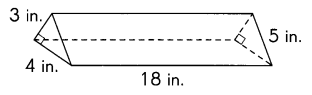Surface area of triangular prism = 228 in.2

Explanation:
2 × $$\frac{1}{2}$$ × 3 in × 4 in = 12 in.2
4 in × 18 in = 72 in.2
3 in × 18 in = 54 in.2
5 in × 18 in = 90 in.2
12 in.2+ 72  in.2 + 54 in.2 + 90 in.2 = 228 in.2
Surface area of triangular prism = 228 in.2

Question 6.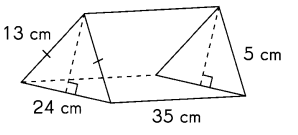Explanation:
The formula for the surface area of triangular prism is:
Surface area = bh + (l X w + w X h + l X h),
= (5 cm X 35 cm) + (13 cm X 24 cm + 24 cm X 5 cm + 13 cm X 35 cm),
= 175  cm2+ ( 312 cm2+ 120 cm2+ 455 cm2)
= 1,062 cm2.

Question 7.

Jeffrey cuts out the net of a box he wants to make.
Find the surface area of the box.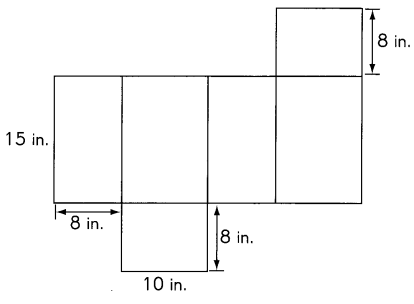The surface area of Jeffrey box is 684 in.2,

Explanation:
Jeffrey box contains 1 square of 8 in side,
2 rectangles of length 15 in and 8 in width,
2 rectangles of length 15 in and 10 in width,
1 rectangle of length 10 in and 8 in width,
So the surface area of Jeffrey box is
8 in X 8 in = 64 in.2
2 X (15 in X 8 in) = 2 X 120 in.2= 240 in.2
2 X (15 in X 10 in) = 2 X 150 in.2= 300 in.2
1 X (10 in X 8 in) = 80 in.2
surface area = 64 in.2+ 240 in.2 + 300 in.2+ 80 in.2,
surface area = 684 in.2.

Question 8.
This glass fish tank does not have a cover. Find the total area of the
glass panels used to make the tank.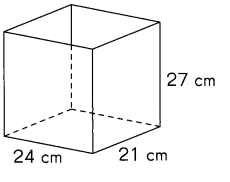The total area of the glass panels used to make the tank is = 288 cm2,

Explanation:
Given glass fish tank that does not have a cover. The total area of the
glass panels used is in the shape of cuboid,
therefore to make the tank we need
2(lw + lh + hw) = 2 X [(24 cm + 21 cm) + (24 cm + 27 cm) + (27 cm + 21 cm)],
= 2 X (45 cm2 + 51 cm2 + 48 cm2),
= 2 X (144 cm2),
= 288 cm2.

Question 9.
The tank shown is made of steel. It does not have a cover.
Find the area of steel sheet used to make the tank.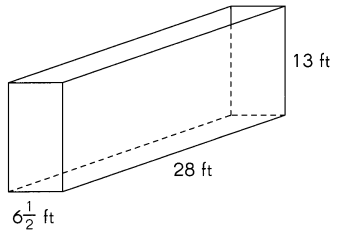The total area of the steel sheet is 190 ft2,

Explanation:
Given tank shown is made of steel,
The total area of the steel sheet used is in the shape of rectangular prism,
therefore to make the tank we need
2(lw + lh + hw) = 2 X [(6 1/2 ft + 28 ft) + (6 1/2 ft + 13 ft) + (13 ft + 28 ft)],
= 2 X (34 1/2 ft2 + 19 1/2 ft2 + 41 ft2),
= 2 X (95 ft2),
= 190 ft2.

Question 10.
A rectangular piece of poster board measures 60 centimeters by 80 centimeters.
Linn draws the net of a box on the poster board and cuts it out.
If the box measures 10 centimeters by 16 centimeters by 27 centimeters,
what is the area of the poster board left?